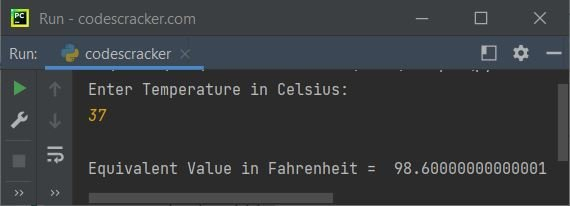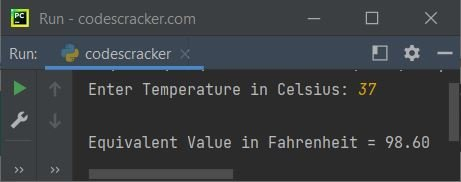# Python Program to Convert Celsius to Fahrenheit

In this article, we've created some programs in Python, to convert a temperature entered by user in Celsius to its equivalent Fahrenheit value. Here are the list of programs:

• Simple Celsius to Fahrenheit Conversion Program
• Convert Celsius to Fahrenheit using Function
• Using class

Note - Before creating these programs, let's first see the formula used for the conversion.

### Celsius to Fahrenheit Formula

The Celsius to Fahrenheit formula is:

`F = (C * 1.8) + 32`

Here C indicates to the value of Celsius entered by user. And F indicates to Fahrenheit value.

## Simple Celsius to Fahrenheit Conversion

To convert temperature from Celsius to Fahrenheit in Python, you have to ask from user to enter the temperature in Celsius, then convert that temperature into its equivalent Fahrenheit value as shown in the program given below:

```print("Enter Temperature in Celsius: ")
cel = float(input())

fah = (cel*1.8)+32
print("\nEquivalent Value in Fahrenheit = ", fah)
```

Here is its sample run:Now enter the temperature value in Celsius say 37 and press `ENTER` key to convert and print its equivalent temperature in Fahrenheit as shown in the snapshot given below:Note - To format output of Fahrenheit value upto only two decimal places, then refer to the program given below.

#### Modified Version of Previous Program

The {:.2f} is used to format any floating-point values upto two decimal places using format() method. The end= is used to skip automatic printing of newline using print()

```print("Enter Temperature in Celsius: ", end="")
cel = float(input())

fah = (cel*1.8)+32
print("\nEquivalent Value in Fahrenheit = {:.2f}" .format(fah))```

Here is its sample run with same user input as of previous program's sample run:## Celsius to Fahrenheit using Function

This program uses a user-defined function named CelToFah() to do the same job as of previous program. That is, the function takes Celsius value as its argument and returns its equivalent Fahrenheit value. Therefore, the returned value gets initialized to fah variable. And the value of fah variable gets printed on output as equivalent Fahrenheit value:

```def CelToFah(c):
return (c*1.8)+32

print("Enter Temperature in Celsius: ", end="")
cel = float(input())

fah = CelToFah(cel)
print("\nEquivalent Value in Fahrenheit = {:.2f}" .format(fah))```

This program produces exactly same output as of previous program.

## Celsius to Fahrenheit using Class

This is the last program created using a class named CodesCracker. Class is an object-oriented feature of Python.

```class CodesCracker:
def CelToFah(self, c):
return (c*1.8)+32

print("Enter Temperature in Celsius: ", end="")
cel = float(input())

ob = CodesCracker()
fah = ob.CelToFah(cel)
print("\nEquivalent Value in Fahrenheit = {:.2f}" .format(fah))```

In this program, an object ob of class CodesCracker() is created to access its member function named CelToFah() using dot (.) operator.

#### Same Program in Other Languages

Python Online Test

« Previous Program Next Program »